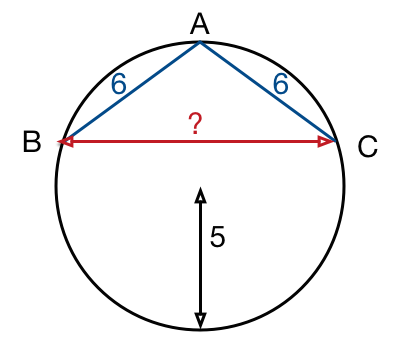# Isosceles Triangle In Circle?

Geometry Level 2In a circle of radius $5 \text{ cm}$, $AB$ and $AC$ are 2 chords such that $AB=AC=6 \text{ cm}$ . the length of chord $BC$ is $\text{\_\_\_\_\_\_\_\_\_\_ cm}$.

×

Problem Loading...

Note Loading...

Set Loading...